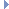﻿ MAtomic Class Reference

# MAtomic Class ReferenceRelated help topics:

`#include <MAtomic.h>`

## Class Description

Methods for atomic operations.

The MAtomic class implements several cross-platform atomic operations which are useful when writing a multithreaded application. Atomic operations are those that appear to happen as a single operation when viewed from other threads.

As a usage example, during reference counting in an SMP environment, it is important to ensure that decrementing and testing the counter against zero happens atomically. If coded this way:

if (–counter == 0) {}

then another thread could modify the value of counter between the decrement and the if test. The above code would therefore get the wrong value. This class provides a routine to perform the decrement and return the previous value atomically, so the above snippet could be written as:

if (MAtomic::preDecrement(&counter) == 0) {}

## Static Public Member Functions

static FORCE_INLINE int preIncrement (volatile int *variable)
Increment variable, return value after increment. More...

static FORCE_INLINE int postIncrement (volatile int *variable)
Increment variable, return value before increment. More...

static FORCE_INLINE int increment (volatile int *variable, int incrementValue)
Increment variable by incrementValue, return value before increment. More...

static FORCE_INLINE int preDecrement (volatile int *variable)
Decrement variable, return value after increment. More...

static FORCE_INLINE int postDecrement (volatile int *variable)
Decrement variable, return value before decrement. More...

static FORCE_INLINE int decrement (volatile int *variable, int decrementValue)
Decrement variable by decrementValue, return value before decrement. More...

static FORCE_INLINE int set (volatile int *variable, int newValue)
Set variable to newValue, return value of variable before set. More...

static FORCE_INLINE int compareAndSwap (volatile int *variable, int compareValue, int swapValue)
Compare variable with compareValue and if the values are equal, sets *variable equal to swapValue. More...

## Member Function Documentation

 static FORCE_INLINE int preIncrement ( volatile int * variable )
inlinestatic

Increment variable, return value after increment.

Parameters
 [in] variable Value to be modified
Returns
. Variable value after incrementing
 static FORCE_INLINE int postIncrement ( volatile int * variable )
inlinestatic

Increment variable, return value before increment.

Parameters
 [in] variable Value to be modified
Returns
. Variable value before incrementing
 static FORCE_INLINE int increment ( volatile int * variable, int incrementValue )
inlinestatic

Increment variable by incrementValue, return value before increment.

Parameters
 [in] variable Value to be modified [in] incrementValue Value by which to increment variable
Returns
. Variable value before incrementingExamples:
 static FORCE_INLINE int preDecrement ( volatile int * variable )
inlinestatic

Decrement variable, return value after increment.

Parameters
 [in] variable Value to be modified
Returns
. Variable value after decrementing
 static FORCE_INLINE int postDecrement ( volatile int * variable )
inlinestatic

Decrement variable, return value before decrement.

Parameters
 [in] variable Value to be modified
Returns
. Variable value before decrementing
 static FORCE_INLINE int decrement ( volatile int * variable, int decrementValue )
inlinestatic

Decrement variable by decrementValue, return value before decrement.

Parameters
 [in] variable Value to be modified [in] decrementValue Value by which to decrement variable
Returns
. Variable value before decrementing
 static FORCE_INLINE int set ( volatile int * variable, int newValue )
inlinestatic

Set variable to newValue, return value of variable before set.

Parameters
 [in] variable Value to be modified [in] newValue Value to which to set variable
Returns
. Variable value before set
 static FORCE_INLINE int compareAndSwap ( volatile int * variable, int compareValue, int swapValue )
inlinestatic

Compare variable with compareValue and if the values are equal, sets *variable equal to swapValue.

The result of the comparison is returned, true if the compare was sucessful, false otherwise.

Parameters
 [in] variable First value to be compared [in] compareValue Second value to be compared [in] swapValue Value to set *variable to if comparison is successful
Returns
. True if variable equals compareValue, otherwise false

The documentation for this class was generated from the following file:
• MAtomic.h